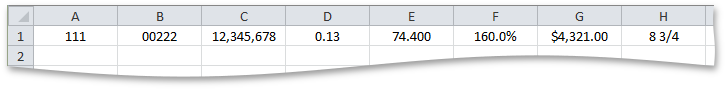21.2
21.1
20.2
20.1
19.2
19.1
18.2
18.1
17.2
.NET Framework 4.5.2+
.NET Framework 4.5.2+
.NET Standard 2.0+

# Formatting.NumberFormat Property

Gets or sets a number format that specifies how a numeric value is displayed in a cell.

## Declaration

``string NumberFormat { get; set; }``

## Property Value

Type Description
String

Specifies a number format in the invariant culture (English).

## Remarks

Numbers can appear in cells as percentage, decimal, currency, accounting, or date time values.The NumberFormat property allows you to use both the built-in and custom number formats. Refer to the How to: Specify Number or Date Format for Cell Content example for details.

## Example

This example demonstrates how to use different number formats to display numeric values in cells.

``````// Display 111 as 111.
worksheet.Cells["A1"].Value = 111;
worksheet.Cells["A1"].NumberFormat = "#####";

// Display 222 as 00222.
worksheet.Cells["B1"].Value = 222;
worksheet.Cells["B1"].NumberFormat = "00000";

// Display 12345678 as 12,345,678.
worksheet.Cells["C1"].Value = 12345678;
worksheet.Cells["C1"].NumberFormat = "#,#";

// Display .126 as 0.13.
worksheet.Cells["D1"].Value = .126;
worksheet.Cells["D1"].NumberFormat = "0.##";

// Display 74.4 as 74.400.
worksheet.Cells["E1"].Value = 74.4;
worksheet.Cells["E1"].NumberFormat = "##.000";

// Display 1.6 as 160.0%.
worksheet.Cells["F1"].Value = 1.6;
worksheet.Cells["F1"].NumberFormat = "0.0%";

// Display 4321 as \$4,321.00.
worksheet.Cells["G1"].Value = 4321;
worksheet.Cells["G1"].NumberFormat = "\$#,##0.00";

// Display 8.75 as 8 3/4.
worksheet.Cells["H1"].Value = 8.75;
worksheet.Cells["H1"].NumberFormat = "# ?/?";
``````